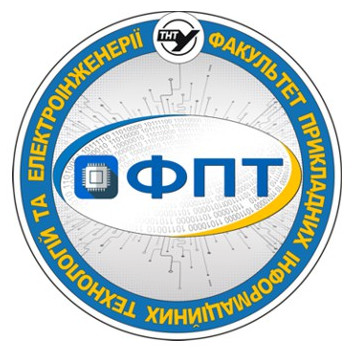## Картка дисципліни

Select major*Ternopil Ivan Puluj National Technical University

Факультет прикладних інформаційних технологій та електроінженерії

Кафедра біотехнічних систем

Biomedical Processes and Signal Modelling

syllabus

 Major 163 - Біомедична інженерія (бакалавр) Field of knowledge 16 Хімічна та біоінженерія Academic degree bachelor's
Course
Course type elective
special education
Study start course 3
Semesters 5
Form of education full-time
Study hours structure
 32 – lectures 32 – laboratory classes
Amount of hours for individual work 56
ECTS credits 4
Form of final examination exam
Lecturer
 Academic degree Cand. Sc. Academic title Assoc. Prof. Full name Шадріна Галина Михайлівна
Prerequirements (prerequisite courses)
 Anatomy and physiology, Higher mathematics, Programming and computer technology
Course goals and learning objectives
 Purpose and objectives of the discipline 1. purpose: to prepare students for the study of complex systems and processes based on mathematical modeling methods. 2. objectives: to reveal the content of the basic concepts, object, methods and principles of modeling; give an idea of the types of modeling and the main hikes to constructing mathematical models of systems; research and optimization of biological processes and systems at different levels of their organization
Course description
 Lectures 1 A Model Concept. A model. A little history. What is mathematical modeling? Why mathematical modeling? Basic numerical tasks. Numerical linear algebra. Numerical analysis. Numerical data analysis. Numerical functional analysis. Non-numerical algorithms. The modeling diagram. What are some limitations of mathematical models? How are mathematical models derived? Model’s adequacy degrees. Some models examples. 2 Some Theoretical Basis of Modeling. Modeling for prediction. Modeling methods. Biological processes and modeling. The modeling process. Validation and verification of the model. 3 Types of Models. Decision in a model designing. Degrees of a mathematical model complexity. Types of models. Computational models. Main computational modeling approaches. Graphs in modeling. Boolean/qualitative networks. Spatio-temporal models. 4 Biosignals and biological processes. What is biosignal? Permanent biosignals. Induced biosignals. Static biosignal. Dynamic biosignal. Classifications of biosignals. 5 Methods of biosignals models creation and processing. Types of signal to be modeled. How to create amodel for your problem. Using the modeling diagram. Constructing block diagram as a human organism state model. Analysis of block diagram. Mathematical modeling. Stages of mathematical modeling. Limitations and advantages of the mathematical modeling method. Basic concepts of digital signal processing. Fourier methods in signal processing. Filtration in signal processing and modeling. General methodology for time-frequency - (t,f) bio-signal analysis. Models as dynamic systems. Continuous models. Discrete models. The parameter problem. Measuring and calculating. 6 An Example of biosignal model creation and analysis. Biosignals learning and synthesis using deep neural networks. Deep neural networks and gated recurrent units. Character-level language model. 7 Electromyographic signals selection and pre-processing for a hand bio-controlled prosthesis. Electromiographic electrodes construction. Experimental results. 8 An electroencephalographic and electromyographic signals mathematical model for a task of a human communicative function renewal. Statement of a problem. Analysis of available investigations. Results of the investigation. Verification of the mathematical model. Conclusions. 9 A human communicative function indirect restoration method. Introduction. A method of human communicative function indirect restoration. Experimental results. 10 Detection of biomedical signals disruption using a sliding window. Introduction. The survey of available investigations. A problem statement. Results of the researches. Conclusion. 11 The speech process signs determination in electroencephalographic signals structure. Introduction. A problem statement. Criterion of the speech process beginning and completion time moments determination using electroencephalographic signals. Evaluation f the EEG signal processing results reliability. Conclusion. 12 Vocal signals mathematical model for the tasks of human vocal apparatus diagnostics. Introduction. Materials and methods. Grounding of a VFS mathematical model. Results. 13 Theoretical basis of phonocardiosignal modeling. A problem formulation. Resent researches analysis. Research results. Conclusions. 14 Twenty four hours pulse signal simulation algorithm. What is a pulse signal. A problem formulation. The twenty-four hours pulse signal structure. The twenty-four hours pulse signal simulation model and algorithm. Software for the twenty four hours pulse signal simulation. Conclusions. 15 How to develop new biosignals based products. What is PLUX. Accessing biosignals. Design and development process. PLUX ‘s methodological approach and toolkits. PLUX approach to design new products based on human biosignals. Quality assessment of biosignals. 16 Analysis of peripheral physiological data. Materials and methods. Electrocardiogram. Electrodermal activity. Electromyography. Continuous blood pressure. Impedance cardiography. Practical classes - Laboratory classes Safety instructions. Introduction to MATLAB. Operators of control of the computing process in the environment of processing of biomedical information. Operation with vectors and matrices. Create non-simple file functions (procedures) in the MATLAB environment. Functions of functions. Graphic design of the results of processing biomedical signals in the form of 2d-graphs. Graphic design of biomedical signal processing results in the form of 3d graphs. Load biosignals and save processing results to a file. Correlation processing of biosignals. Spectral processing of biosignals. Spectral correlation processing of biosignals. Statistical processing of biosignals. Wavelet processing of biosignals. Single-phase processing. Component method for processing biosignals. Final discussion.
Assessment criteria
 Criteria of students’ progress assessment Module 1 – 40 points: 10 points – test, 30 points – lab works. Module 2 – 35 points: 10 points – test, 25 points – lab works. Form of final control – examination – max 25 points